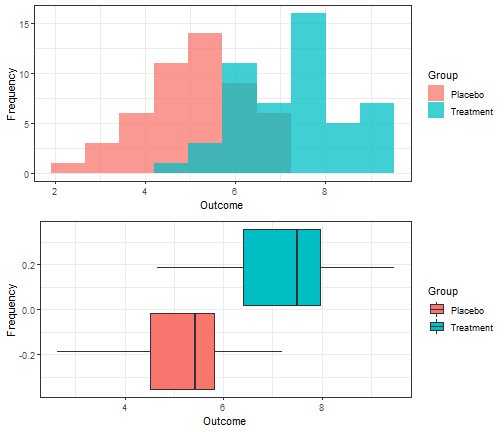## Plug-in estimators of statistical functionals

Consider a sequence of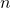independent and identically distributed random variables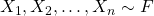. The distribution function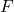is unknown but belongs to a known set of distribution functions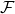. In parametric estimation,may represent a family of distributions specified by a vector of parameters, such as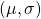in the case of the location-scale family. In nonparametric estimation,is much more broad and is subject to milder restrictions, such as the existence of moments or continuity. For example, we may defineas the family of distributions for which the mean exists or all distributions defined on the real line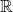.

As mentioned in my previous blog post comparing nonparametric and parametric estimation, a statistical functional is any real-valued function of the cumulative distribution function, denoted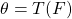. Statistical functionals can be thought of as characteristics of, and include moments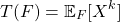and quantiles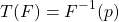as examples.

An infinite population may be considered as completely determined by its distribution function, and any numerical characteristic of an infinite population with distribution functionthat is used in statistics is a [statistical] functional of.

Wassily Hoeffding. “A Class of Statistics with Asymptotically Normal Distribution.” Ann. Math. Statist. 19 (3) 293 – 325, September, 1948.

This blog post aims to provide insight into estimators of statistical functionals based on a sample ofindependent and identically random variables, known as plug-in estimators or empirical functionals.

## Using a DAG to simulate data with the dagR library

Directed acyclic graphs (DAGs), and causal graphs in general, provide a framework for making assumptions explicit and identifying confounders or mediators of the relationship between the exposure of interest and outcome that need to be adjusted for in analysis. Recently, I ran into the need to generate data from a DAG for a paper I am writing with my peers Kevin McIntyre and Joshua Wiener. After a quick Google search, I was pleasantly surprised to see there were several options to do so. In particular, the dagR library provides “functions to draw, manipulate, [and] evaluate directed acyclic graphs and simulate corresponding data”.

Besides dagR‘s reference manual, a short letter published in Epidemiology, and a limited collection of examples, I couldn’t find too many resources regarding how to use the functionality provided by dagR. The goal of this blog post is to provide an expository example of how to create a DAG and generate data from it using the dagR library.

To simulate data from a DAG with dagR, we need to:

1. Create the DAG of interest using the dag.init function by specifying its nodes (exposure, outcome, and covariates) and their directed arcs (directed arrows to/from nodes).
2. Pass the DAG from (1) to the dag.sim function and specify the number of observations to be generated, arc coefficients, node types (binary or continuous), and parameters of the node distributions (Normal or Bernoulli).

For this tutorial, we are going to try to replicate the simple confounding/common cause DAG presented in Figure 1b as well as the more complex DAG in Figure 2a of Shier and Platt’s (2008) paper, Reducing bias through directed acyclic graphs.

library(dagR)
set.seed(12345)

## Parametric vs. Nonparametric Approach to Estimations

Parametric statistics assume that the unknown CDFbelongs to a family of CDFs characterized by a parameter (vector)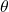. As the form ofis assumed, the target of estimation is its parameters. Thus, all uncertainty aboutis comprised of uncertainty about its parameters. Parameters are estimated by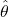, and estimates are be substituted into the assumed distribution to conduct inference for the quantities of interest. If the assumed distributionis incorrect, inference may also be inaccurate, or trends in the data may be missed.

To demonstrate the parametric approach, consider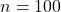independent and identically distributed random variables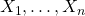generated from an exponential distribution with rate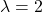. Investigators wish to estimate the 75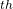percentile and erroneously assume that their data is normally distributed. Thus,is assumed to be the Normal CDF but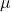and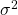are unknown. The parametersand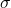are estimated in their typical way by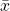and, respectively. Since the normal distribution belongs to the location-scale family, an estimate of the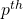percentile is provided by,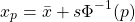where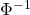is the standard normal quantile function, also known as the probit.

set.seed(12345)
library(tidyverse, quietly = T)

# Generate data from Exp(2)
x <- rexp(n = 100, rate = 2)

# True value of 75th percentile with rate = 2
true <- qexp(p = 0.75, rate = 2)
true

##  0.6931472

# Estimate mu and sigma
xbar <- mean(x)
s    <- sd(x)

# Estimate 75th percentile assuming mu = xbar and sigma = s
param_est <- xbar + s * qnorm(p = 0.75)
param_est

##  0.8792925


The true value of the 75percentile of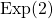is 0.69 while the parametric estimate is 0.88.

Nonparametric statistics make fewer distributions about the unknown distribution, requiring only mild assumptions such as continuity or the existence of specific moments. Instead of estimating parameters of,itself is the target of estimation.is commonly estimated by the empirical cumulative distribution function (ECDF)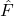,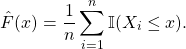Any statistic that can be expressed as a function of the CDF, known as a statistical functional and denoted, can be estimated by substitutingfor. That is, plug-in estimators can be obtained as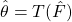.

## The Probabilistic Index for Two Normally Distributed Outcomes

Consider a two-armed study comparing a placebo and treatment. In general, the probabilistic index (PI) is defined as,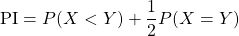and is interpreted as the probability that a subject in the treatment group will have an increased response compared to a subject in the placebo group. The probabilistic index is a particularly useful effect measure for ordinal data, where effects can be difficult to define and interpret owing to absence of a meaningful difference. However, it can also be used for continuous data, noting that when the outcome is continuous,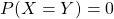and the PI reduces to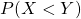.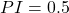suggests an increased outcome is equally likely for subjects in the placebo and treatment group, while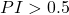suggests an increased outcome is more likely for subjects in the treatment group compared to the placebo group, and the opposite is true when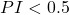.

## Simulation

Suppose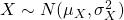and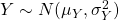represent the independent outcomes in the placebo and treatment groups, respectively and an increased value of the outcome is the desired response.

We simulate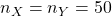observations from each group such that treatment truly increases the outcome and the variances within each group are equal such that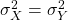.

# Loading required libraries
library(tidyverse)
library(gridExtra)

# Setting seed for reproducibility
set.seed(12345)

# Simulating data
n_X = n_Y = 50
sigma_X = sigma_Y = 1
mu_X = 5; mu_Y = 7

outcome_X = rnorm(n = n_X, mean = mu_X, sd = sigma_X)
outcome_Y = rnorm(n = n_Y, mean = mu_Y, sd = sigma_Y)

df <- data.frame(Group = c(rep('Placebo', n_X), rep('Treatment', n_Y)),
Outcome = c(outcome_X, outcome_Y))


Examining side-by-side histograms and boxplots of the outcomes within each group, there appears to be strong evidence that treatment increases the outcome as desired. Thus, we would expect a probabilistic index close to 1 as most outcomes in the treatment group appear larger than those of the placebo group.

# Histogram by group
hist_p <- df %>%
ggplot(aes(x = Outcome, fill = Group)) +
geom_histogram(position = 'identity', alpha = 0.75, bins = 10) +
theme_bw() +
labs(y = 'Frequency')

# Boxplot by group
box_p <- df %>%
ggplot(aes(x = Outcome, fill = Group)) +
geom_boxplot() +
theme_bw() +
labs(y = 'Frequency')

# Combine plots
grid.arrange(hist_p, box_p, nrow = 2)